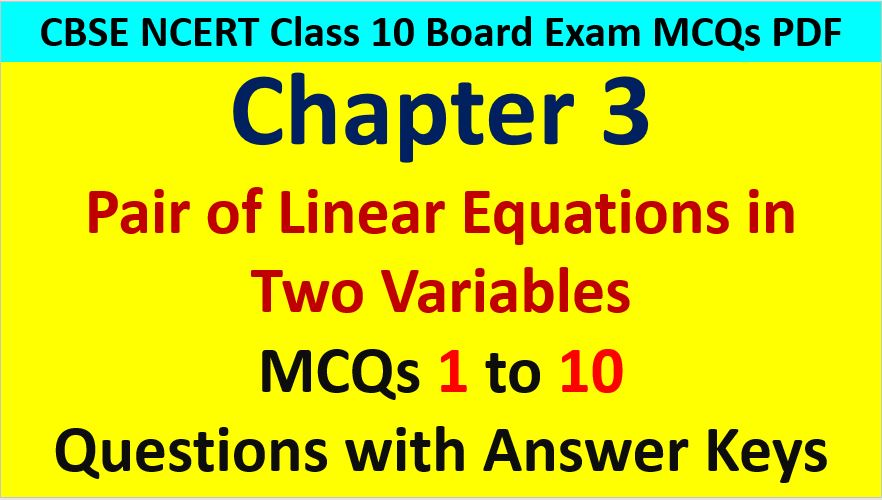Friday, October 22, 2021
Home > CBSE Class 10 > MCQ Questions for Class 10 Maths Chapter 3 Pair of Linear Equations in Two Variables

# MCQ Questions for Class 10 Maths Chapter 3 Pair of Linear Equations in Two VariablesHi students, Welcome to AMBiPi (Amans Maths Blogs). In this article, you will get MCQ Questions for Class 10 Maths Chapter 3 Pair of Linear Equations in Two Variables with Answer Keys PDF. You can download this PDF and save it in your mobile device or laptop etc.

MCQ Questions for Class 10 Maths Linear Equations in Two Variables Question No 1:

Customers are asked to stand in the lines. If one customer is extra in a line, then there would be two less lines. If one customer is less in line, there would be three more lines. Find the number of students in the class.

Option A : 40

Option B : 50

Option C : 60

Option D : 70

Option C : 60

MCQ Questions for Class 10 Maths Linear Equations in Two Variables Question No 2:

A pair of linear equations a1x + b1y + c1 = 0; a2x + b2y + c2 = 0 is said to be inconsistent, if

Option A : a1/a2 ≠ b1/b2

Option B : a1/a2 = b1/b≠ c1/c2

Option C : a1/a2 = b1/b= c1/c2

Option D : a1/a2 ≠ c1/c2

Option B : a1/a2 = b1/b≠ c1/c2

MCQ Questions for Class 10 Maths Linear Equations in Two Variables Question No 3:

Graphically, the pair of equations : 6x – 3y + 10 = 0 and 2x – y + 9 = 0. Represents two lines which are

Option A : Intersecting at exactly one point

Option B : Intersecting at exactly two points.

Option C : Coincident

Option D : Parallel

Option D : Parallel

MCQ Questions for Class 10 Maths Linear Equations in Two Variables Question No 4:

Graphically, the pair of equations 7x – y = 5; 21x – 3y = 10 represents two lines which are

Option A : intersecting at one point

Option B : parallel

Option C : intersecting at two points

Option D : coincident

Option B : Parallel

MCQ Questions for Class 10 Maths Linear Equations in Two Variables Question No 5:

The pairs of equations x+2y-5 = 0 and -4x-8y+20=0 have:

Option A : Unique solution

Option B : Exactly two solutions

Option C : Infinitely many solutions

Option D : No solution

Option C : Infinitely Many Solutions

MCQ Questions for Class 10 Maths Linear Equations in Two Variables Question No 6:

If a pair of linear equations is consistent, then the lines will be

Option A : always coincident

Option B : parallel

Option C : always intersecting

Option D : Intersecting or coincident

Option D : intersecting or coincident

MCQ Questions for Class 10 Maths Linear Equations in Two Variables Question No 7:

The pair of equations x = 0 and x = 5 has

Option A : no solution

Option B : unique/one solution

Option C : two solutions

Option D : infinitely many solutions

Option C : Two Solutions

MCQ Questions for Class 10 Maths Linear Equations in Two Variables Question No 8:

The pair of equation x = – 4 and y = – 5 graphically represents lines which are

Option A : intersecting at (- 5, – 4)

Option B : intersecting at (- 4, – 5)

Option C : intersecting at (5, 4)

Option D : intersecting at (4, 5)

Option B : intersecting at (-4, -5)

MCQ Questions for Class 10 Maths Linear Equations in Two Variables Question No 9:

The pair of equations 6x – 10y = 7 and – 6x + 10y = 9 have

Option A : a unique solution

Option B : infinitely many solutions

Option C : no solution

Option D : two solution

Option C : No Solutions

MCQ Questions for Class 10 Maths Linear Equations in Two Variables Question No 10:

Assertion : Homogenous system of linear equations is always consistent.

Reason : x = 0 and y = 0 is always a solution of homogenous system of linear equation with unknown x and y.

Which of the following statement is true?

Option A : Assertion is true and Reason is correct explanation of Assertion.

Option B : Assertion is false and Reason is NOT correct explanation of Assertion.

Option C : Assertion is true and Reason is false.

Option D : Assertion is false and Reason is true.